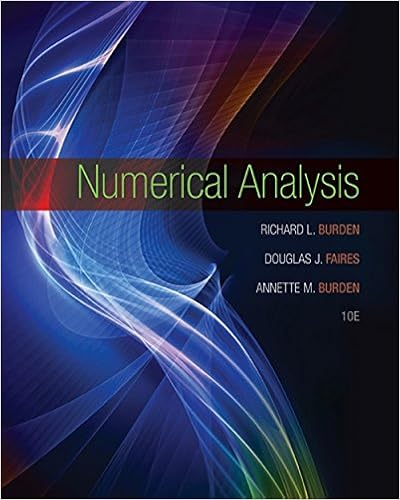# Homework 4 Solutions - STAT 225 Fall 2016-Homework...

• Homework Help
• 5
• 100% (5) 5 out of 5 people found this document helpful

This preview shows page 1 - 2 out of 5 pages.

##### We have textbook solutions for you!
The document you are viewing contains questions related to this textbook.The document you are viewing contains questions related to this textbook.
Chapter 4 / Exercise 20
Numerical Analysis
Burden/FairesExpert Verified
STAT 225 Fall 2016--Homework 4 ---SOLUTIONS. 1.The average life of particular type of light bulb used at a university is 8,000 hours. There are 10 light bulbs in a classroom. In order for a class to be held in the room, 7 or more light bulbs must be working. Each light bulb works independently of the other light bulbs in a classroom. a)Name the distribution of a light bulb’s lifeand specify the parameters.
1
b)What is the probability that a single light bulb works for over 8,000 hours?
?
c)Given a light bulb has already operated for 5,000 hours, what’s the probability that it will operate more than 11,000 hours total?
?
d)Given a light bulb operates no more than 12,000 hours, what’s the probability that it breaks before 7,000 hours?
e)Assuming that all the light bulbs in a room were installed at the same time, what is the probability that a class can be held in that room after 8,000 hours of the light bulbsoperation? ? Let X be the number of working light bulbs 8,000 hours or more. X ~ Binomial(n=12, p= 0.3679) note: p comes from prob of
+
2.X is a random variable with a probability density function given by a)Determine the value of cto make this a valid probability density function.
?
3
2
##### We have textbook solutions for you!
The document you are viewing contains questions related to this textbook.The document you are viewing contains questions related to this textbook.
Chapter 4 / Exercise 20
Numerical Analysis
Burden/FairesExpert Verified
•••